2022 NFL Comeback Player of the Year Odds
+400
4 to 1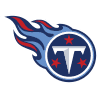Derrick Henry
11.6% implied probability

+550
5.5 to 1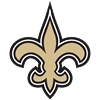Jameis Winston
9.0% implied probability

+750
7.5 to 1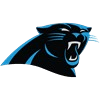Christian McCaffrey
6.8% implied probability

+800
8 to 1Michael Thomas
6.5% implied probability

+800
8 to 1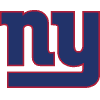Daniel Jones
6.5% implied probability

+1000
10 to 1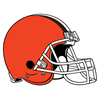Deshaun Watson
5.3% implied probability

+1200
12 to 1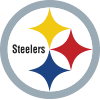Mitch Trubisky
4.5% implied probability

+1200
12 to 1Baker Mayfield
4.5% implied probability

+1400
14 to 1Chase Young
3.9% implied probability

+1400
14 to 1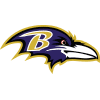J.K. Dobbins
3.9% implied probability

+1600
16 to 1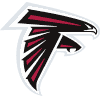Marcus Mariota
3.4% implied probability

+2500
25 to 1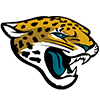Travis Etienne
2.2% implied probability

+2500
25 to 1Cam Akers
2.2% implied probability

+2500
25 to 1Allen Robinson
2.2% implied probability

+2500
25 to 1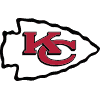JuJu Smith-Schuster
2.2% implied probability

+3000
30 to 1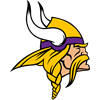Danielle Hunter
1.9% implied probability

+4000
40 to 1Chris Godwin
1.4% implied probability

+4000
40 to 1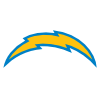Khalil Mack
1.4% implied probability

+4000
40 to 1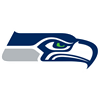Drew Lock
1.4% implied probability

+5000
50 to 1Will Fuller
1.1% implied probability

+5000
50 to 1Odell Beckham
1.1% implied probability

No changes have been recorded yet.
+5000
50 to 1Robert Woods
1.1% implied probability

+5000
50 to 1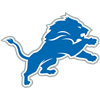Jeff Okudah
1.1% implied probability

No changes have been recorded yet.
+5000
50 to 1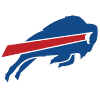Tre'Davious White
1.1% implied probability

+5000
50 to 1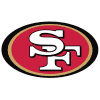Trey Lance
1.1% implied probability

No changes have been recorded yet.
+5000
50 to 1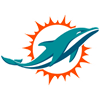Raheem Mostert
1.1% implied probability

+5000
50 to 1Marcus Peters
1.1% implied probability

+5000
50 to 1James White
1.1% implied probability

+5000
50 to 1J.J. Watt
1.1% implied probability

+6000
60 to 1Carl Lawson
1.0% implied probability

+8000
80 to 1DeAndre Hopkins
0.7% implied probability

+8000
80 to 1DJ Chark
0.7% implied probability

+8000
80 to 1Blake Martinez
0.7% implied probability

+10000
100 to 1Jaycee Horn
0.6% implied probability

+10000
100 to 1Gus Edwards
0.6% implied probability

+10000
100 to 1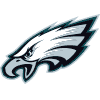Brandon Graham
0.6% implied probability

+10000
100 to 1Dan Arnold
0.6% implied probability

+10000
100 to 1Marlon Humphrey
0.6% implied probability

+10000
100 to 1Ronnie Stanley
0.6% implied probability

+10000
100 to 1Maxx Williams
0.6% implied probability

+10000
100 to 1Darrell Henderson
0.6% implied probability